• matlab开发-高斯正态分布概率密度函数。高斯正态分布是应用最广泛的分布之一。
• 正态分布概率密度函数的积分
正态分布概率密度函数的积分

I

=

∫

−

∞

∞

1

2

π

σ

e

−

(

x

−

μ

)

2

2

σ

2

d

x

\begin{aligned} I = \int _{- \infty} ^{\infty} \frac{1}{\sqrt{2 \pi} \sigma} e ^ {- \frac{(x - \mu) ^2}{2 \sigma ^ 2}} dx \end{aligned}

指数中包含二次项，无法直接求解，需要做一些变换。
夹逼定理

F

(

x

)

,

G

(

x

)

F(x), G(x)

在

x

0

x_0

连续且极限相同,即

lim

⁡

x

→

x

0

F

(

x

)

=

lim

⁡

x

→

x

0

G

(

x

)

=

A

\begin{aligned} \lim_{x \to x_0}F(x) = \lim_{x \to x_0}G(x) = A \end{aligned}

f

(

x

)

f(x)

在

x

0

x_0

的某领域内恒有

F

(

x

)

≤

f

(

x

)

≤

G

(

x

)

F(x) \le f(x) \le G(x)

则

lim

⁡

x

→

x

0

f

(

x

)

=

A

\begin{aligned} \lim_{x \to x_0}f(x) = A \end{aligned}

二重积分的换元法

∬

x

2

+

y

2

≤

R

2

f

(

x

,

y

)

d

x

d

y

=

∫

0

R

∫

0

2

π

f

(

r

s

i

n

θ

,

r

c

o

s

θ

)

r

d

r

d

θ

\begin{aligned} &\iint_{x^2+y^2 \le R^2} f(x, y)dxdy \\ = &\int_0^R \int_0^{2\pi} f(rsin\theta, rcos\theta) \ r \ dr d\theta \end{aligned}

正态分布概率密度函数的积分
(1) 令

y

=

x

−

μ

2

σ

\begin{aligned} y = \frac{x - \mu}{\sqrt{2} \sigma} \end{aligned}

, 得到

I

=

1

π

∫

−

∞

∞

e

−

y

2

d

y

\begin{aligned} I = \frac {1}{\sqrt{\pi}} \int _{-\infty} ^{\infty} e ^ {- y^2} dy \end{aligned}

(2) 令

U

=

∫

−

∞

∞

e

−

y

2

d

y

\begin{aligned} U = \int _{-\infty} ^{\infty} e ^ {- y^2} dy \end{aligned}

, 则

I

=

1

π

U

\begin{aligned} I = \frac {1}{\sqrt{\pi}} U \end{aligned}

(3)

U

=

lim

⁡

R

→

∞

∫

−

R

R

e

−

y

2

d

y

\begin{aligned} U = \lim_{R \to \infty} \int _{-R} ^{R} e ^ {- y^2} dy \end{aligned}

(4) 转换为正方形区域内的二重积分

U

2

=

lim

⁡

R

→

∞

∫

−

R

R

e

−

x

2

d

x

∫

−

R

R

e

−

y

2

d

y

=

lim

⁡

R

→

∞

∫

−

R

R

∫

−

R

R

e

−

x

2

−

y

2

d

x

d

y

=

lim

⁡

R

→

∞

∬

−

R

≤

x

≤

R

,

−

R

≤

y

≤

R

e

−

x

2

−

y

2

d

x

d

y

\begin{aligned} U^2 &= \lim_{R \to \infty} \int _{-R} ^{R} e ^ {- x^2} dx \int _{-R} ^{R} e ^ {- y^2} dy \\ &= \lim_{R \to \infty} \int _{-R} ^{R}\int _{-R} ^{R} e ^ {- x^2-y^2} dx dy \\ &= \lim_{R \to \infty} \iint _{-R \le x \le R, -R \le y \le R} e ^ {- x^2-y^2} dx dy \end{aligned}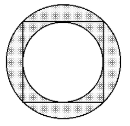(5) 内切圆积分

U

1

=

lim

⁡

R

→

∞

∬

x

2

+

y

2

≤

R

2

e

−

x

2

−

y

2

d

x

d

y

=

lim

⁡

R

→

∞

∫

0

R

∫

0

2

π

e

−

r

2

r

d

r

d

θ

=

∫

0

2

π

d

θ

×

lim

⁡

R

→

∞

∫

0

R

e

−

r

2

r

d

r

=

2

π

∫

0

∞

e

−

r

2

r

d

r

=

2

π

(

−

1

2

e

−

r

2

∣

0

∞

)

=

π

\begin{aligned} U1 &= \lim_{R \to \infty} \iint _{x^2+y^2 \le R^2} e ^ {- x^2-y^2} dx dy \\ &= \lim_{R \to \infty} \int _{0}^{R} \int_{0}^{2 \pi} e ^ {- r^2} r \ dr d\theta \\ &= \int_{0}^{2 \pi} d\theta \times \lim_{R \to \infty} \int _{0}^{R} e ^ {- r^2} r \ dr \\ &= 2 \pi \int _{0}^{\infty} e ^ {- r^2} r \ dr \\ &= 2 \pi (- \frac{1}{2} e ^ {- r^2} |_0^\infty) \\ &= \pi \end{aligned}

(5) 外接圆积分

U

2

=

lim

⁡

R

→

∞

∬

x

2

+

y

2

≤

2

R

2

e

−

x

2

−

y

2

d

x

d

y

=

lim

⁡

R

→

∞

∫

0

2

R

∫

0

2

π

e

−

r

2

r

d

r

d

θ

=

∫

0

2

π

d

θ

×

lim

⁡

R

→

∞

∫

0

2

R

e

−

r

2

r

d

r

=

2

π

∫

0

∞

e

−

r

2

r

d

r

=

2

π

(

−

1

2

e

−

r

2

∣

0

∞

)

=

π

\begin{aligned} U2 &= \lim_{R \to \infty} \iint _{x^2+y^2 \le 2R^2} e ^ {- x^2-y^2} dx dy \\ &= \lim_{R \to \infty} \int _{0}^{\sqrt{2}R} \int_{0}^{2 \pi} e ^ {- r^2} r \ dr d\theta \\ &= \int_{0}^{2 \pi} d\theta \times \lim_{R \to \infty} \int _{0}^{\sqrt{2}R} e ^ {- r^2} r \ dr \\ &= 2 \pi \int _{0}^{\infty} e ^ {- r^2} r \ dr \\ &= 2 \pi (- \frac{1}{2} e ^ {- r^2} |_0^\infty) \\ &= \pi \end{aligned}

(6)

e

−

x

2

−

y

2

e ^ {- x^2-y^2}

在整个平面上都大于0，因此有

∬

x

2

+

y

2

≤

R

2

e

−

x

2

−

y

2

d

x

d

y

<

∬

−

R

≤

x

≤

R

,

−

R

≤

y

≤

R

e

−

x

2

−

y

2

d

x

d

y

<

∬

x

2

+

y

2

≤

2

R

2

e

−

x

2

−

y

2

d

x

d

y

\begin{aligned} &\iint _{x^2+y^2 \le R^2} e ^ {- x^2-y^2} dx dy \\ \lt &\iint _{-R \le x \le R, -R \le y \le R} e ^ {- x^2-y^2} dx dy \\ \lt &\iint _{x^2+y^2 \le 2R^2} e ^ {- x^2-y^2} dx dy \end{aligned}

夹逼定理可得

U

2

=

U

1

=

U

2

=

π

U^2 = U1 = U2 = \pi

因此

U

=

π

,

I

=

1

π

U

=

1

\begin{aligned}U=\sqrt{\pi}, I = \frac {1}{\sqrt{\pi}} U = 1 \end{aligned}


展开全文• 本文首先给出正态分布概率密度函数（The normal distribution probability density function）的公式和标准正态分布概率密度函数的公式，然后通过normpdf( )生成标准正态分布概率密度函数的数据，然后通过plot( )...
本文首先给出正态分布概率密度函数（The normal distribution probability density function）的公式和标准正态分布概率密度函数的公式，然后通过normpdf( )生成标准正态分布概率密度函数的数据，然后通过plot( )绘制标准正态分布概率密度函数的图形。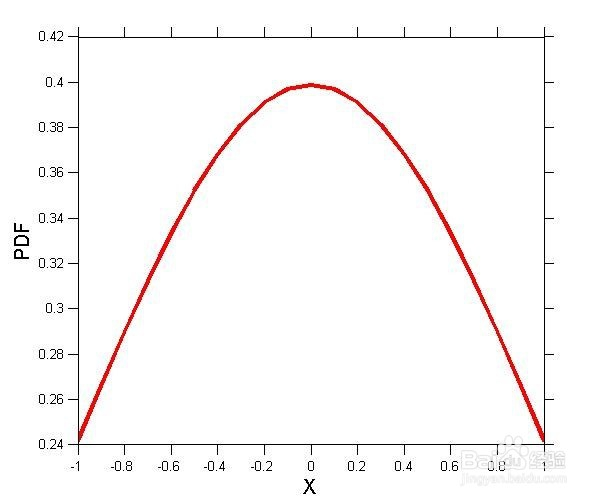工具/原料
MATLAB  normpdf  mean  standard deviation
方法/步骤
第一，正态分布概率密度函数的公式如下图。其中，μ为平均值（mean），σ为标准差（standard deviation）。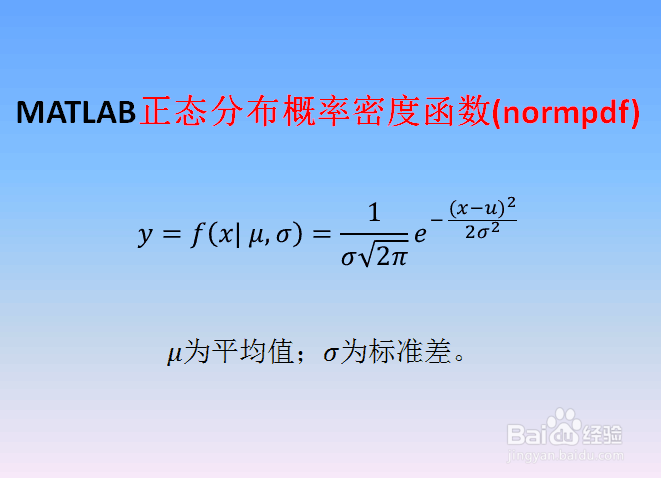第二，当μ=0，σ=1时，第一步中的正态分布为标准正态概率密度函数，如下图。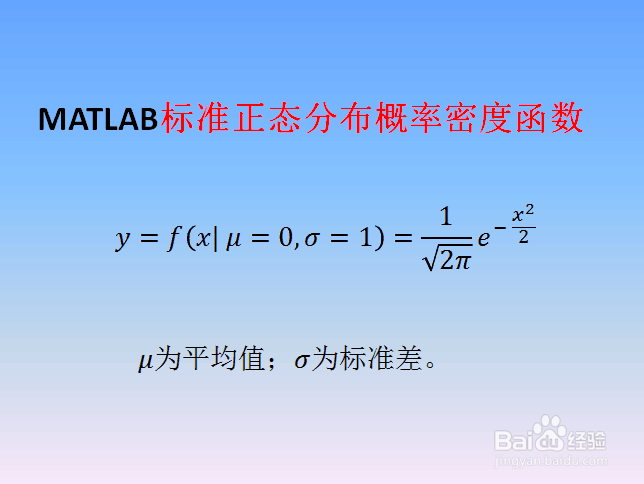第三，启动MATLAB，新建脚本（Ctrl+N），输入如下代码： close all; clear all; clc x=-1:.1:1; norm=normpdf(x,0,1); figure('Position',[50,50,600,500],'Name','Normal PDF',...     'Color',[1,1,1]); plot(x,norm,'r-','LineWidth',3) set(gca,'FontSize',10,'TickDir','out','TickLength',[0.02,0.02]) xlabel('X','FontSize',15);ylabel('PDF','FontSize',15) 其中normpdf(x,0,1)是用来产生μ=0，σ=1的标准正态分布概率密度函数的数据。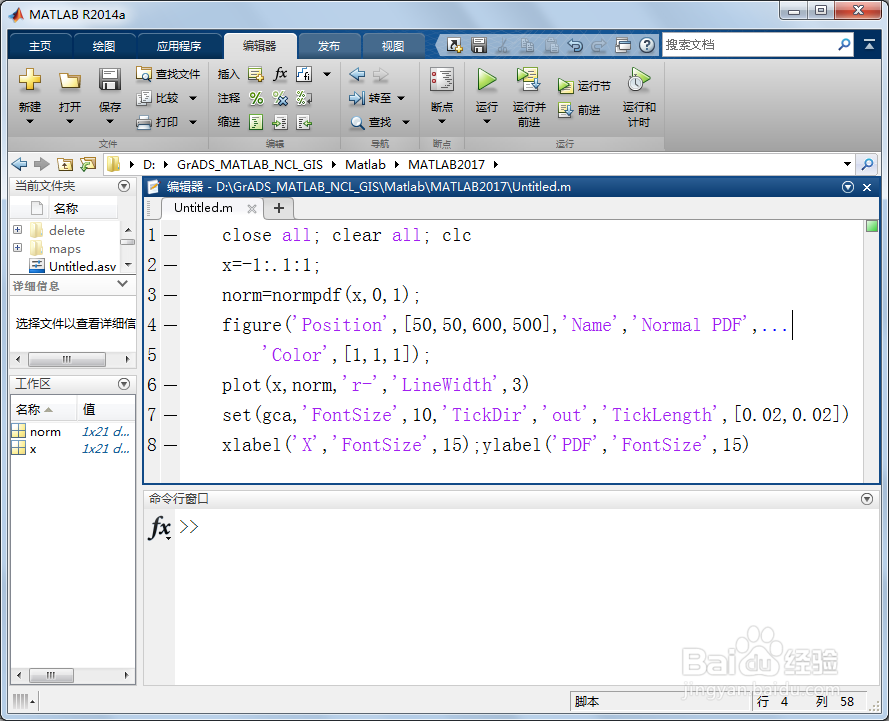第四，保存和运行上述脚本，在工作区（Workspace）得到标准正态分布概率密度函数的数据norm，双击norm可以查看数据具体内容。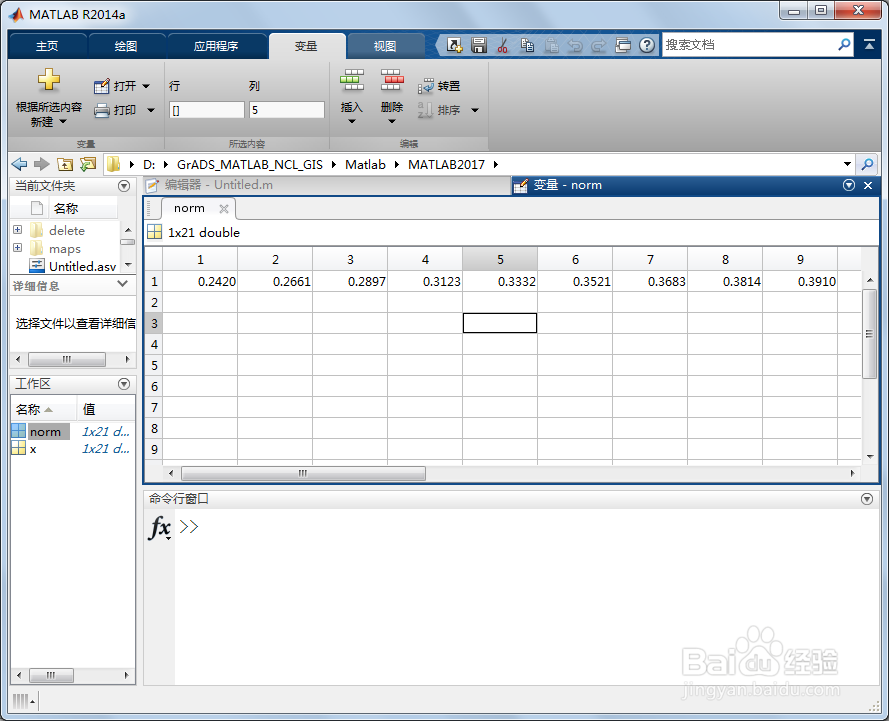第五，同时得到如下μ=0，σ=1的标准正态分布概率密度函数图形。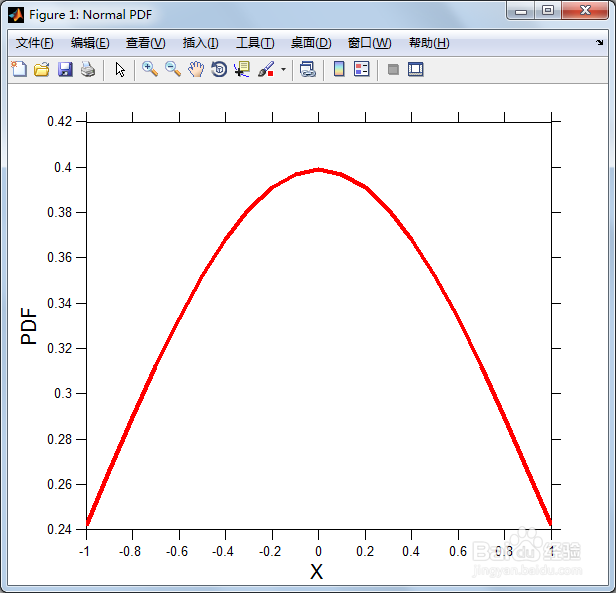展开全文• 行业分类-物理装置-一种基于正态分布概率密度函数的互联网交易平台不确定性评价滤波系统.zip
• 这是我整理的关于gamma函数及用gamma函数证明标准正态分布概率密度函数的归一性的一点内容，传上来希望对大家有所帮助。
• 正态分布概率密度函数均值为μ 方差为σ2 (或标准差σ)是高斯函数的一个实例： 。 (请看指数函数以及π.) 如果一个随机变量X服从这个分布，我们写作 X ~ N(μ,σ2). 如果μ = 0
概率密度函数，这种方法能够表示随机变量每个取值有多大的可能性。

概率密度函数四个不同参数集的概率密度函数（绿色线代表标准正态分布）

正态分布的概率密度函数均值为μ 方差为σ2 (或标准差σ)是高斯函数的一个实例：$f(x;\mu,\sigma)=\frac{1}{\sigma\sqrt{2\pi}} \, \exp \left( -\frac{(x- \mu)^2}{2\sigma^2} \right)$。

(请看指数函数以及π.)
如果一个随机变量X服从这个分布，我们写作 X ~ N(μ,σ2). 如果μ = 0并且σ = 1，这个分布被称为标准正态分布，这个分布能够简化为$f(x) = \frac{1}{\sqrt{2\pi}} \, \exp\left(-\frac{x^2}{2} \right)$。

右边是给出了不同参数的正态分布的函数图。
正态分布中一些值得注意的量：
密度函数关于平均值对称平均值是它的众数（statistical mode）以及中位数（median）函数曲线下68.268949%的面积在平均值左右的一个标准差范围内95.449974%的面积在平均值左右两个标准差2σ的范围内99.730020%的面积在平均值左右三个标准差3σ的范围内99.993666%的面积在平均值左右四个标准差4σ的范围内反曲点（inflection point）在离平均值的距离为标准差之处

累积分布函数上图所示的概率密度函数的累积分布函数

累积分布函数是指随机变量X小于或等于x的概率，用密度函数表示为$F(x;\mu,\sigma)=\frac{1}{\sigma\sqrt{2\pi}}\int_{-\infty}^x \exp \left( -\frac{(x - \mu)^2}{2\sigma^2}\ \right)\, dx.$

正态分布的累积分布函数能够由一个叫做误差函数的特殊函数表示：$\Phi(z)=\frac12 \left[1 + \mathrm{erf}\,(\frac{z-\mu}{\sigma\sqrt2})\right] .$

标准正态分布的累积分布函数习惯上记为Φ，它仅仅是指μ = 0，σ = 1时的值，$\Phi(x)=F(x;0,1)=\frac{1}{\sqrt{2\pi}}\int_{-\infty}^x\exp\left(-\frac{x^2}{2}\right)\, dx.$

将一般正态分布用误差函数表示的公式简化，可得：$\Phi(z)=\frac{1}{2} \left[ 1 + \operatorname{erf} \left( \frac{z}{\sqrt{2}} \right) \right].$

它的反函数被称为反误差函数，为：$\Phi^{-1}(p)=\sqrt2\;\operatorname{erf}^{-1} \left(2p - 1 \right).$

该分位数函数有时也被称为probit函数。probit函数已被证明没有初等原函数。
正态分布的分布函数Φ(x)没有解析表达式，它的值可以通过数值积分、泰勒级数或者渐进序列近似得到。

性质

正态分布的一些性质:
如果$X \sim N(\mu, \sigma^2) \,$且a与b是实数，那么aX + b∼N(aμ + b,(aσ)2) (参见期望值和方差).如果$X \sim N(\mu_X, \sigma^2_X)$与$Y \sim N(\mu_Y, \sigma^2_Y)$是统计独立的正态随机变量，那么:
它们的和也满足正态分布$U = X + Y \sim N(\mu_X + \mu_Y, \sigma^2_X + \sigma^2_Y)$ (proof).它们的差也满足正态分布$V = X - Y \sim N(\mu_X - \mu_Y, \sigma^2_X + \sigma^2_Y)$.U与V两者是相互独立的。 如果$X \sim N(0, \sigma^2_X)$和$Y \sim N(0, \sigma^2_Y)$是独立正态随机变量，那么:
它们的积XY服从概率密度函数为p的分布$p(z) = \frac{1}{\pi\,\sigma_X\,\sigma_Y} \; K_0\left(\frac{|z|}{\sigma_X\,\sigma_Y}\right),$其中K0是贝塞尔函数（modified Bessel function）

它们的比符合柯西分布，满足X / Y∼Cauchy(0,σX / σY). 如果$X_1, \cdots, X_n$为独立标准正态随机变量，那么$X_1^2 + \cdots + X_n^2$服从自由度为n的卡方分布。

展开全文• 这里是一个简单的实现代码 x=linspace（-5,5,50); %生成负五到五之间的五十个数，行矢量 y=normpdf(x,0,1); plot(x,y,‘k’);...第一，正态分布概率密度函数的公式如下图。其中，μ为平均值（mean...
这里是一个简单的实现代码 x=linspace（-5,5,50); %生成负五到五之间的五十个数，行矢量 y=normpdf(x,0,1); plot(x,y,‘k’); 图片复制不过来。。就摆个链接好了 https://jingyan.baidu.com/article/6fb756ec70be3f241858fbe2.html 第一，正态分布概率密度函数的公式如下图。其中，μ为平均值（mean），σ为标准差（standard deviation）。2第二，当μ=0，σ=1时，第一步中的正态分布为标准正态概率密度函数，如下图。3第三，启动MATLAB，新建脚本（Ctrl+N），输入如下代码：close all; clear all; clcx=-1:.1:1;norm=normpdf(x,0,1);figure(‘Position’,[50,50,600,500],‘Name’,‘Normal PDF’,… ‘Color’,[1,1,1]);plot(x,norm,‘r-’,‘LineWidth’,3)set(gca,‘FontSize’,10,‘TickDir’,‘out’,‘TickLength’,[0.02,0.02])xlabel(‘X’,‘FontSize’,15);ylabel(‘PDF’,‘FontSize’,15)其中normpdf(x,0,1)是用来产生μ=0，σ=1的标准正态分布概率密度函数的数据。4第四，保存和运行上述脚本，在工作区（Workspace）得到标准正态分布概率密度函数的数据norm，双击norm可以查看数据具体内容。5第五，同时得到如下μ=0，σ=1的标准正态分布概率密度函数图形。
展开全文• 概率密度函数 概率密度函数（PDF）。 随机变量的（PDF）为 其中mu是平均值，而sigma > 0是标准偏差。 安装 \$ npm install distributions-normal-pdf 要在浏览器中使用，请使用 。 用法 var pdf = require ( '...JavaScript
• miu = 0 sigma = 1 x = np.linspace(miu - 3 * sigma, miu + 3 * sigma, 50) y = np.exp(-(x - miu) ** 2 / (2 * sigma ** 2)...plt.title('高斯分布函数', fontsize=18) plt.grid(True) plt.show()matplotlib
• 看概率统计书上都是直接给出正态分布概率密度函数，有时候好奇为什么要是这个样子，于是上网查了一下，发现其是可以通过一些合理的前提假定推导出来的。 链接如下： ...数学基础
• 最近利用碎片时间在读Allen B.Downey的《贝叶斯思维：统计建模的Python学习法》，顺便用手机上的Pythonista写实例。...累积分布函数（Cumulative Distribution Function，CDF）就是概率密度函数（Probability De...
• 目录1、scipy库中各分布对应的方法2、stats中各分布的常用方法及其功能3、正态分布概率密度函数及其图象1）正态分布概率密度函数及其图象2）python绘制正态分布概率密度函数图象4、卡方分布的概率密度函数及其...
• plt.suptitle('Gaussian PDF', fontsize=16) plt.tight_layout() plt.show() 以上这篇python高斯分布概率密度函数的使用详解就是小编分享给大家的全部内容了，希望能给大家一个参考，也希望大家多多支持我们。...
• python 服从正态分布概率密度函数和累积密度函数 服从正太分布下,概率密度函数公式 公式解释: f(x): 是某样本(样本以数值形式表现)为某数值时发生的概率 0<f(x)<1 x: 是随机抽样的数值,取值范围从负...python
• 二元正态分布随机变量 如果随机变量XXX、YYY的联合PDF为 pX,Y(x,y)=12πσxσY1−p2exp⁡{−(x−μX)2σX2+(y−μY)2σY2−2ρ(x−μX)(y−μY)σXΣY2(1−ρ2)}p_{X,Y}(x,y)=\frac{1}{2\pi \sigma_x \sigma_Y \...
• 正态分布概率密度函数入手,利用导数研究函数,推导正态分布的大致图像和基本性质.
• Excel图表—标准正态分布概率分布图（概率密度函数图及累积概率分布图）的绘制.pdf
• 正态分布 函数形式：y=normpdf(x,mu,sigma) x - x轴数据，如 x=-10:0.01:10; 注意正态分布，正负对称！ mu - 均值，默认为0； sigma - 标准差，默认为1； 实例 x=-10:0.01:10;y=normpdf(x,0,1);plot(x,y); ...
• 首先，已知有一个满足一维正态分布概率密度函数为： p(x) = exp{} 在多元正态分布下，其数据为x =，对应的均值方差分别为 =，=， 则其概率密度可由一下展开表示： p(x) = =exp{} *exp{} *exp{}...exp{} ...人工智能
• 计算概率分布的相关参数时，一般使用 scipy 包，常用的函数包括以下几个：pdf：连续随机分布概率密度函数pmf：离散随机分布概率密度函数cdf：累计分布函数百分位函数（累计分布函数的逆函数）生存函数的逆函数...
• <p>r语言画二维正态分布概率密度曲线的代码是什么呀</p>有问必答 r语言
• 绘制二元正态分布密度函数图和等高线图，mesh函数（密度图）和contour函数（等高线）MATLAB
• 在MATLAB中使用 Y = normpdf(X,MU,SIGMA)函数求一维正态分布概率密度，其中X为随机矢量，MU为期望，SIGMA为标准差 matlab代码如下： x=-8:0.1:8; y1=normpdf(x,0,1);%期望为0，标准差为1的正态分布 y2=normpdf...matlab 模式识别
• 正太分布和概率密度函数，期望值，方差 正态分布(Normal distribution)，又名高斯分布（Gaussian distribution）是一个非常常见的连续概率分布。正态分布在统计学上十分重要，经常用在自然和社会科学来代表一个不明...统计学
• normcdf函数用来获得正态分布的概率分布函数； 也就是 normcdf(x)=Pr{Z≤x}Pr...若想获得均值为 μ\mu，方差为 σ\sigma的概率分布函数：normcdf(x,mu,sigma)即可.normpdf用来获得正态分布概率密度函数. 用法与上同.matlab
• parzen窗的matlab实现 本文档实现的是用简单的matlab程序实现parzen窗的设计parzen
• python 用 pandas通过样本计算出均值和方差，得到正态分布密度函数 ** 求：P（add）*P（add|key）*p(add|key.min&gt;=1) import pandas as pd import math data=[] with open(r’D:\666.txt’,‘r’)as f: for...python 贝叶斯 pandas...